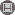دوره 8، شماره 1 - ( 2-1392 )                   جلد 8 شماره 1 صفحات 15-29 | برگشت به فهرست نسخه ها

BibTeX | RIS | EndNote | Medlars | ProCite | Reference Manager | RefWorks
Send citation to:Borzooei R, Zahiri O. Radical and It’s Applications in BCH-Algebras. IJMSI. 2013; 8 (1) :15-29
URL: http://ijmsi.ir/article-1-399-fa.html
Radical and It’s Applications in BCH-Algebras. مجله علوم ریاضی و انفورماتیک. 1392; 8 (1) :15-29

چکیده:

Let \$X\$ be a \$BCH\$-algebra and \$I\$ be an ideal of \$X\$. In this paper, we introduce the concept of \$sqrt{I}\$. We show that it is an ideal of \$X\$, when \$I\$ is closed ideal of \$X\$. Then we verify some useful properties of it. We prove that it is the ::::union:::: of all \$k-\$nil ideals of \$I\$. Moreover, if \$I\$ is a closed ideal of \$X\$, then \$sqrt{I}\$ is a closed translation ideal and so we can construct a quotient \$BCH\$-algebra. We prove this quotient is a P-semisimple \$BCI\$-algebra and so it is an abelian group. Then we use the concept of radical in order to construct the second and the third isomorphism theorems.

نوع مطالعه: پژوهشي | موضوع مقاله: عمومى

ارسال نظر درباره این مقاله : نام کاربری یا پست الکترونیک شما:کلیه حقوق این وب سایت متعلق به نشریه علوم ریاضی و انفورماتیک می باشد.

طراحی و برنامه نویسی : یکتاوب افزار شرق